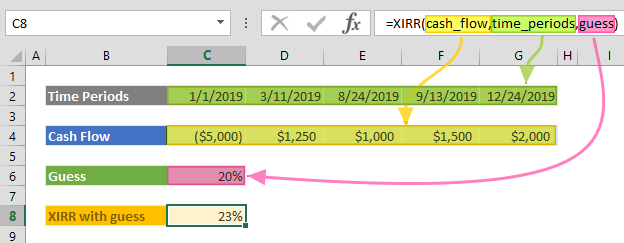The XIRR function is a finance function that calculates the internal rate of return (IRR) of a series of non-periodic cash flows. This function works like the IRR function, but doesn't require entering regular intervals. In this guide, we’re going to show you how to use the XIRR function and also go over some tips and error handling methods.

# Supported versions

• All Excel versions

# Syntax

XIRR(values, dates, [guess])

# Arguments

 values A series of cash flows. Cash flows must contain at least one positive value and one negative value. Payments are expressed as negative values and income as positive. dates Date series of cash flows. The first payment date indicates the beginning of the schedule of payments. All other dates must be later than this date, but they may occur in any order. [guess] Optional. Your estimation for expected internal rate of return. Default is 0.1 (10%).

Examples

Note that we've used named ranges in this example to make the formulas easier to read. This is not required.

## Example 1

=XIRR(cash_flow,time_periods)
formula demonstrates a use case for the XIRR function. It calculates an investment's internal rate of return after four irregular intervals. Subsequent payments are discounted based on a 365-day calendar year. Payments are expressed as negative values and income as positive.

When the [guess] argument is omitted, it will be defaulted as 0.1 and will start the iterations from this value. The XIRR calculates iterations until it reaches a result within 0.00001% accuracy.## Example 2

=XIRR(cash_flow,time_periods,guess)
formula is an example of using the [guess] argument in the XIRR function. You can see the result is equal to the sample that omits the [guess] parameter. In most cases you do not need to enter a [guess] argument. Use different values if you get a #NUM! error.# Tips

• The XIRR function can work with cash flows occurring at irregular intervals. Use the IRR function for periodic cash flows.
• The rate of return is calculated by XIRR is the interest rate corresponding to a 0 (zero) net present value (NPV). Using the return of the XIRR in the XNPV function returns 1.79E-09, which is equal to 0 (zero) within the accuracy of the XIRR function. Sample formula:
=XNPV(XIRR(cash_flow,time_periods,guess),cash_flow,time_periods)
• The function ignores text, logical values, or empty cells.

# Issues

## #NUM!

• If the function cannot find any results within 0.00001% accuracy, or in 100 iterations, you will get a #NUM! error. Try changing the [guess] parameter.
• Any date value can entered that is before than the starting date.
• References of values and date series may have different sizes.
• Cash flows must contain at least one positive value and one negative value.

## #VALUE!

• If an argument is non-numeric, you will get a #VALUE! error.
• If you entered invalid dates, you will get a #VALUE! error.